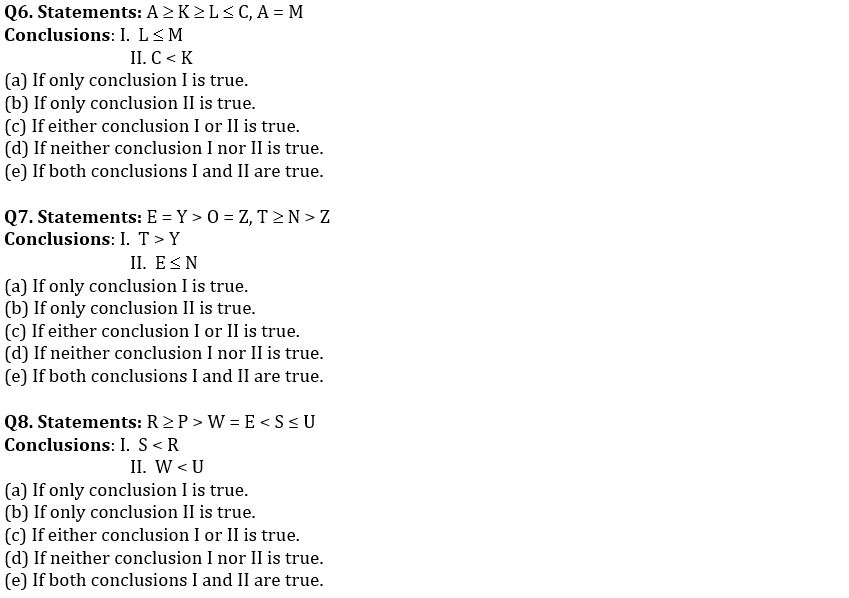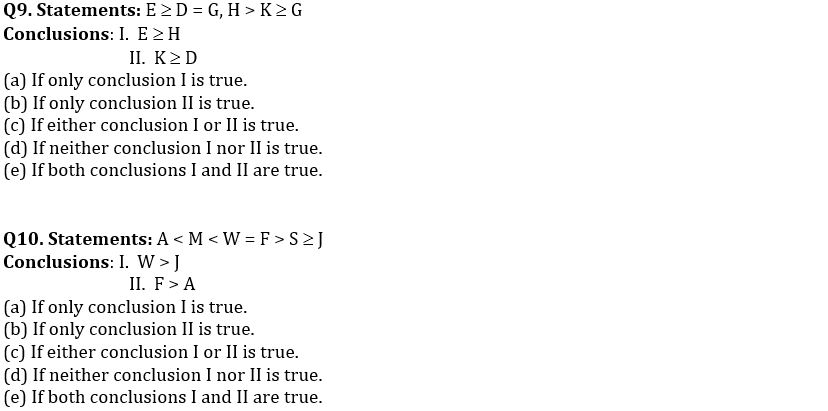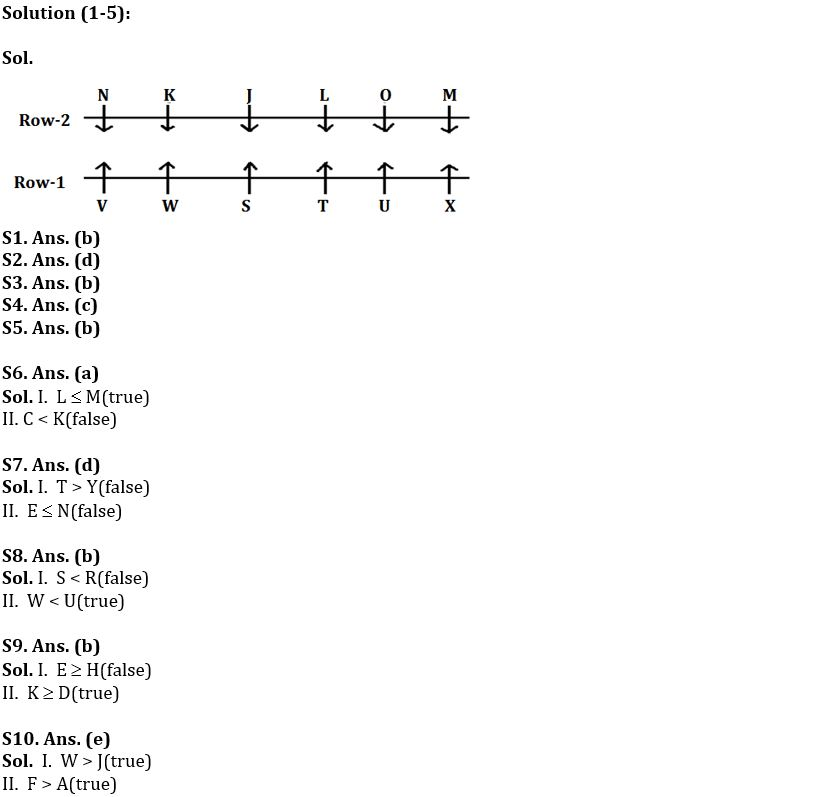Latest Banking jobs   »

# Reasoning Ability Quiz For Bank Foundation 2023- 25th January

Directions (1-5): Study the following information carefully and answer the given questions:
Twelve players are sitting in two parallel rows each containing six players in such way that they are equidistant from each other. In row 1- S, T, U, V, W and X are sitting and facing North. In row 2- J, K, L, M, N and O are sitting and facing South, but not necessarily in the same order. Each player who sits in first row faces another player of the second row.
Three players sit between U and V. The one who faces V sits third to the right of L. M faces the one who sits third to the right of S but does not face U. Immediate neighbor of K faces S. Only one player sits between J and N, who faces the one who sits at the immediate left of W. Neither W nor X faces L. O does not sit at any of the extreme ends.

Q1. Who among the following sits between N and J?
(a) L
(b) K
(c) O
(d) M
(e) None of these

Q2. Who among the following faces W?
(a) J
(b) M
(c) N
(d) K
(e) O

Q3. Who among the following sits to the immediate left of O?
(a) N
(b) M
(c) J
(d) K
(e) None of these

Q4. Four of the following five belong to a group in a certain way, which among the following does not belong to that group?
(a) V
(b) N
(c) U
(d) M
(e) X

Q5. How many players sit to the right of T?
(a) Five
(b) Two
(c) One
(d) Four
(e) Three

Directions (6-10): In these questions, a relationship between different elements is shown in the statements. The statements are following by two conclusions. Give answerDirection (11-15): Study the following data carefully and answer the questions accordingly.

629 439 857 596 948

Q11. If one is subtracted from the third digit of each number and then the first and the second digits are interchanged, then which of the following will be the second lowest number?
(a) 629
(b) 948
(c) 596
(d) 439
(e) None of these

Q12. If in each number, all digits are arranged in decreasing order within the number, then which number is the lowest after rearrangement?
(a) 439
(b) 948
(c) 596
(d) 857
(e) None of these

Q13. If in each number, all the digits are arranged in increasing order within the number then which number is the highest after rearrangement?
(a) 596
(b) 857
(c) 948
(d) 629
(e) None of these

Q14. If the first and the third digits in each of the numbers are interchanged, then which of the following will be the second digit of the lowest number?
(a) 2
(b) 3
(c) 9
(d) 4
(e) None of these

Q15. If half of the second lowest number is subtracted from the lowest number, then what will be the result?
(a) 156
(b) 141
(c) 231
(d) 220
(e) None of these

SolutionsS11 Ans. (d)
Sol. After interchange – 268 348 586 955 497
So, second lowest number = 348. So, 439 is the answer.

S12. Ans. (d)
Sol. After rearrangement – 962 943 875 965 984
So, 857 is lowest number.

S13. Ans. (b)
Sol. After rearrangement – 269 349 578 569 489

S14. Ans. (c)
Sol. After interchange – 926 934 758 695 849
So, 9 will be the second digit of the lowest number.

S15. Ans. (b)
Sol. Result = 439 – 596/2 = 141.## FAQs

### What is the selection process of the Bank Clerk?

The selection process of the Bank Clerk is Prelims & Mains.

#### Congratulations!Union Budget 2023-24: Free PDF